## CSTR

exd2
Model of a continuous stirred tank reactor (CSTR).

Variables and parameters:

Da: Damköhler number (bifurcation parameter: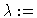Da)

y1 , y2: describe the material and energy balances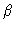: the heat transfer coefficient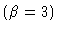B: the rise in adiabatic temperature (B=16.2)

CSTR model equations: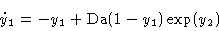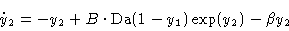Figure 1
Branching diagram y1(0) versus Da. One branch of stationary solutions, and two branches of periodic orbits branching off at two Hopf bifurcations.

Figure 2
y1(t) simulation for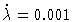,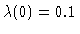,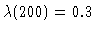with initial values y1(0)=0.1644, y2(0)=0.6658

Figure 3
y1(t) simulation for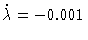,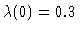,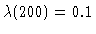with initial values y1(0)=0.9279, y2(0)=3.76

This Example
Figure 1
Figure 2
Figure 3